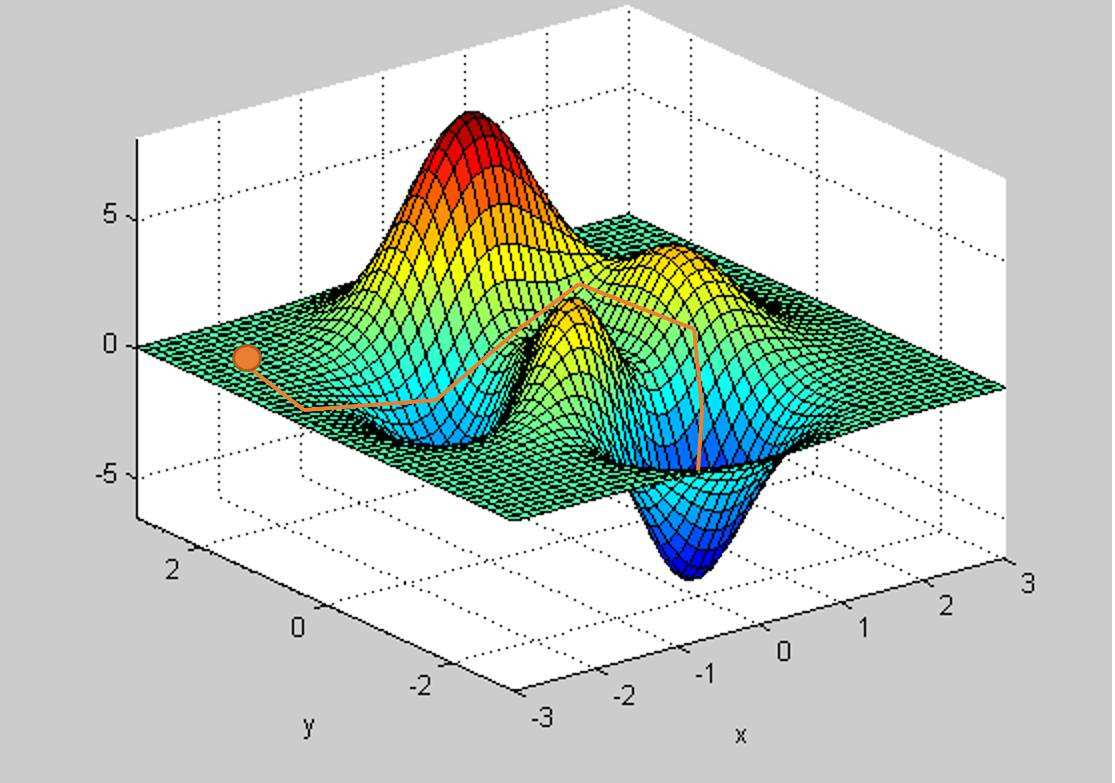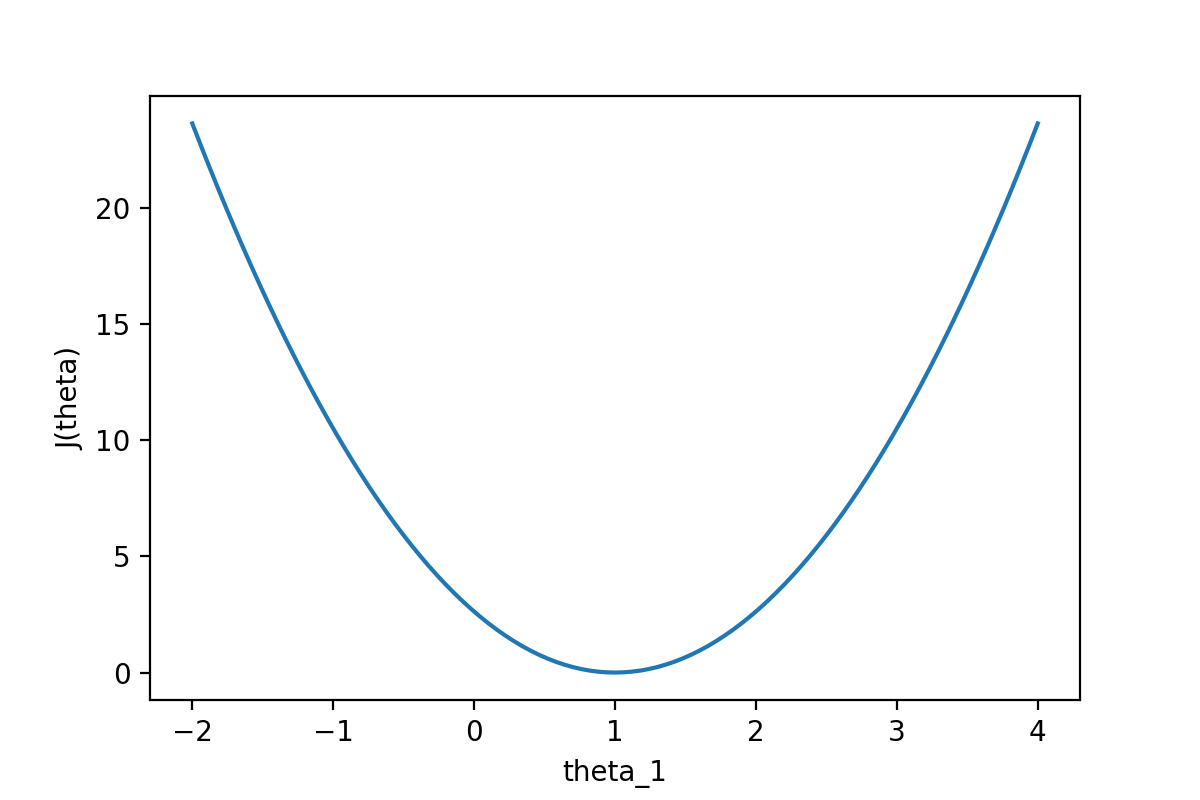# 梯度下降法基本推导### 导言

$$y=ax+b$$

$$\begin{equation} \begin{cases} 69=30a+b \\ 195=90a+b \end{cases} \end{equation}$$

from sympy import *

a,b = symbols("a b")
s1 = solve([Eq(69,30*a+b),Eq(195,90*a+b)],[a,b])
print("a=",s1[a].evalf(),"    b=",s1[b].evalf())


a= 2.10000000000000     b= 6.00000000000000


### 未知数无限多的方程

$$y = a_1x_1+a_2x_2+a_3x_3+ ... +a_{150528}x_{150528}+b$$

$$y = a_1x_1+a_2x_2+a_3x_3+ ... +a_nx_n+b$$

\begin{align} y &= a_0x_0+a_1x_1+a_2x_2+a_3x_3+ ... +a_nx_n \\ &= \sum_{i=0}^na_ix_i \end{align}

### 假设函数

$$y' = h_a(x) = \sum_{i=0}^na_ix_i$$

$$y' = h_θ(x) = \sum_{i=0}^nθ_ix_i$$

### 损失函数

$$l(θ) = (y' - y)^2 = (h_θ(x) - y)^2$$

l是损失(loss)的缩写。这里的差值再取平方就是大名鼎鼎的“方差”，是为了保证结果是一个正数。因为我们肯定希望这个“损失”值越小越好，最好是0，0表示无差别。如果出现了负数，那负的越多，结果实际上更恶劣了。

$$J(θ) = \frac1m\sum_{i=1}^m(h_θ(x^{(i)}) - y^{(i)})^2$$

$$J(θ) = \frac1{2m}\sum_{i=1}^m(h_θ(x^{(i)}) - y^{(i)})^2$$

### 梯度下降求解$$θ_j := θ_j - α\frac∂{∂θ_j}J(θ)$$

\begin{align} \frac∂{∂θ_j}J(θ) & = \frac∂{∂θ_j}\frac1{2m}\sum_{i=1}^m(h_θ(x^{(i)}) - y^{(i)})^2 \\ 化简后 \\ & = \frac1m\sum_{i=1}^m(h_θ(x^{(i)}) - y^{(i)})x_j^i \end{align}

6月24日补充：上面公式化简的过程主要是导数的计算，另外写了一篇详细推导，有需要可自行前往阅读。

$$\begin{cases} θ_0 := θ_0 - α\frac1m\sum_{i=1}^m(h_θ(x^{(i)}) - y^{(i)})^i \\ θ_1 := θ_1 - α\frac1m\sum_{i=1}^m(h_θ(x^{(i)}) - y^{(i)})x_1^i \\ θ_2 := θ_2 - α\frac1m\sum_{i=1}^m(h_θ(x^{(i)}) - y^{(i)})x_2^i \\ ...... \\ θ_j := θ_j - α\frac1m\sum_{i=1}^m(h_θ(x^{(i)}) - y^{(i)})x_j^i \\ θ_n := θ_n - α\frac1m\sum_{i=1}^m(h_θ(x^{(i)}) - y^{(i)})x_n^i \\ \end{cases}$$ 上面公式第一行还记得$$x_0$$恒定为1吗？所以那里并没有$$x_0$$。随后有多少个要求解的θ，就有多少行公式。 从数学角度上说，这些公式没有疑问。从编程上说，有一个提醒。那就是这些公式在求取权重系数θ的时候，每一行求取一个新的θ的过程中，所使用计算假设函数的θ，是在上一个循环中固定下来的那个θ值，所有行的θ均为如此。直到所有这一个批次的θ值计算完成后，整体θ才可以代入到公式，从而参与下一个循环的计算。不能每计算一个θ值，就单独的代入到公式中，那样梯度下降就永远找不到方向了。

### 总结